# SHAP Decision Plots¶

SHAP decision plots show how complex models arrive at their predictions (i.e., how models make decisions). This notebook illustrates decision plot features and use cases with simple examples. For a more descriptive narrative, click here.

For most of the examples, we empoy a LightGBM model trained on the UCI Adult Income data set. The objective: predict whether an individual makes over $50K per year. In : from pprint import pprint import lightgbm as lgb import matplotlib.pyplot as plt import numpy as np import pickle import shap from sklearn.model_selection import train_test_split, StratifiedKFold import warnings X, y = shap.datasets.adult() X_display, y_display = shap.datasets.adult(display=True) # create a train/test split random_state = 7 X_train, X_test, y_train, y_test = train_test_split(X, y, test_size=0.2, random_state=random_state) d_train = lgb.Dataset(X_train, label=y_train) d_test = lgb.Dataset(X_test, label=y_test) params = { "max_bin": 512, "learning_rate": 0.05, "boosting_type": "gbdt", "objective": "binary", "metric": "binary_logloss", "num_leaves": 10, "verbose": -1, "min_data": 100, "boost_from_average": True, "random_state": random_state } model = lgb.train(params, d_train, 10000, valid_sets=[d_test], early_stopping_rounds=50, verbose_eval=1000)  Training until validation scores don't improve for 50 rounds. Early stopping, best iteration is:  valid_0's binary_logloss: 0.277144  ## Calculate SHAP values¶ Compute SHAP values and SHAP interaction values for the first 20 test observations. In : explainer = shap.TreeExplainer(model) expected_value = explainer.expected_value if isinstance(expected_value, list): expected_value = expected_value print(f"Explainer expected value: {expected_value}") select = range(20) features = X_test.iloc[select] features_display = X_display.loc[features.index] with warnings.catch_warnings(): warnings.simplefilter("ignore") shap_values = explainer.shap_values(features) shap_interaction_values = explainer.shap_interaction_values(features) if isinstance(shap_interaction_values, list): shap_interaction_values = shap_interaction_values  Explainer expected value: -2.4296968952292404  # Basic decision plot features¶ Refer to the decision plot of the 20 test observations below. Note: This plot isn't informative by itself; we use it only to illustrate the primary concepts. • The x-axis represents the model's output. In this case, the units are log odds. • The plot is centered on the x-axis at explainer.expected_value. All SHAP values are relative to the model's expected value like a linear model's effects are relative to the intercept. • The y-axis lists the model's features. By default, the features are ordered by descending importance. The importance is calculated over the observations plotted. This is usually different than the importance ordering for the entire dataset. In addition to feature importance ordering, the decision plot also supports hierarchical cluster feature ordering and user-defined feature ordering. • Each observation's prediction is represented by a colored line. At the top of the plot, each line strikes the x-axis at its corresponding observation's predicted value. This value determines the color of the line on a spectrum. • Moving from the bottom of the plot to the top, SHAP values for each feature are added to the model's base value. This shows how each feature contributes to the overall prediction. • At the bottom of the plot, the observations converge at explainer.expected_value. In : shap.decision_plot(expected_value, shap_values, features_display)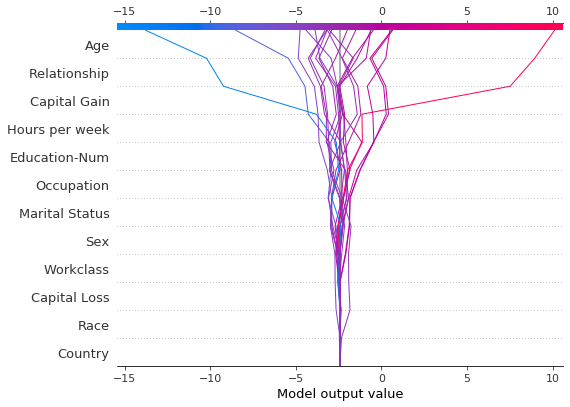Like the force plot, the decision plot supports link='logit' to transform log odds to probabilities. In : shap.decision_plot(expected_value, shap_values, features_display, link='logit')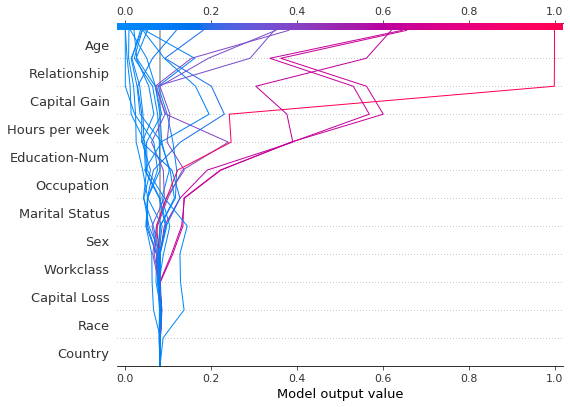Observations can be highlighted using a dotted line style. Here, we highlight a misclassified observation. In : # Our naive cutoff point is zero log odds (probability 0.5). y_pred = (shap_values.sum(1) + expected_value) > 0 misclassified = y_pred != y_test[select] shap.decision_plot(expected_value, shap_values, features_display, link='logit', highlight=misclassified)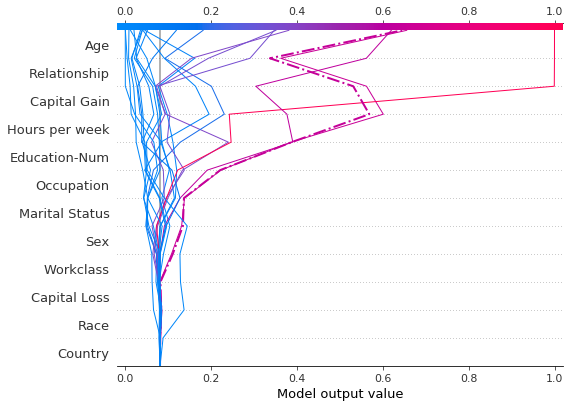Let's inspect the misclassified observation by plotting it alone. When a single observation is plotted, its corresponding feature values are displayed. Notice that the shape of the line has changed. Why? The feature order has changed on the y-axis based on the feature importance for this lone observation. The section "Preserving order and scale between plots" shows how to use the same feature order for multiple plots. In : shap.decision_plot(expected_value, shap_values[misclassified], features_display[misclassified], link='logit', highlight=0)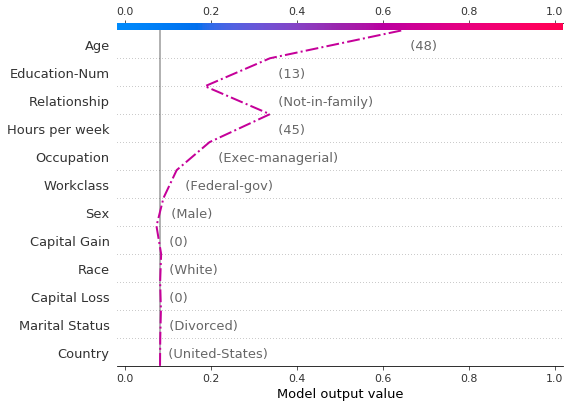A force plot for the misclassified observation is shown below. In this case, the decision plot and the force plot are both effective at showing how the model arrived at its decision. In : shap.force_plot(expected_value, shap_values[misclassified], features_display[misclassified], link='logit', matplotlib=True)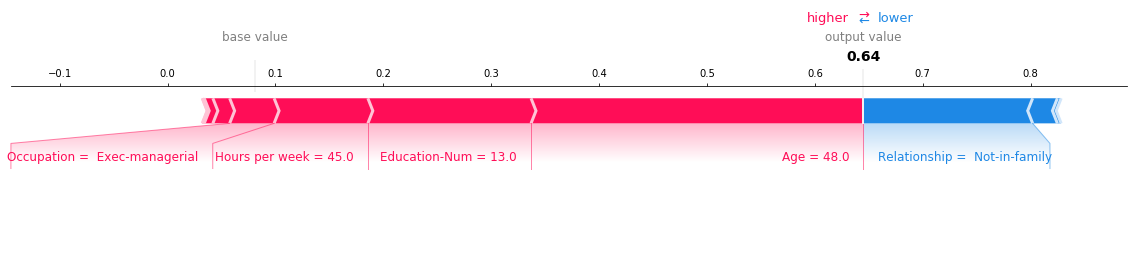# When is a decision plot helpful?¶ There are several use cases for a decision plot. We present several cases here. 1. Show a large number of feature effects clearly. 2. Visualize multioutput predictions. 3. Display the cumulative effect of interactions. 4. Explore feature effects for a range of feature values. 5. Identify outliers. 6. Identify typical prediction paths. 7. Compare and contrast predictions for several models. ## Show a large number of feature effects clearly¶ Like a force plot, a decision plot shows the important features involved in a model's output. However, a decision plot can be more helpful than a force plot when there are a large number of significant features involved. To demonstrate, we use a model trained on the UCI Communities and Crime data set. The model uses 101 features. The two plots below describe the same prediction. The force plot's horizontal format prevents it from showing all of the significant features clearly. In contrast, the decision plot's vertical format can display the effects of any number of features. In : # Load the prediction from disk to keep the example short. with open('./data/crime.pickle', 'rb') as fl: a, b, c = pickle.load(fl) shap.force_plot(a, b, c, matplotlib=True)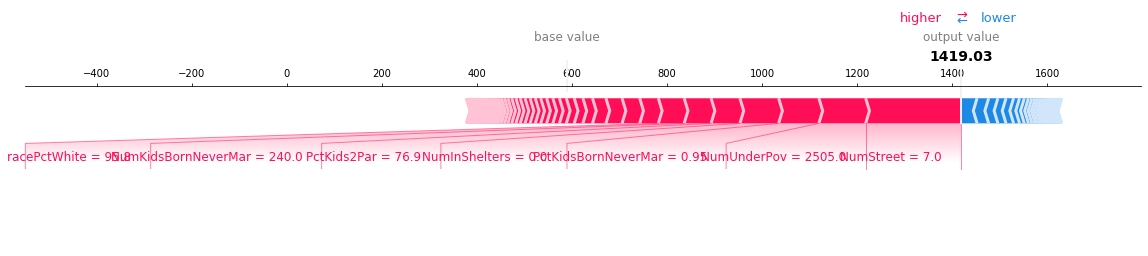In : shap.decision_plot(a, b, c, feature_display_range=slice(None, -31, -1))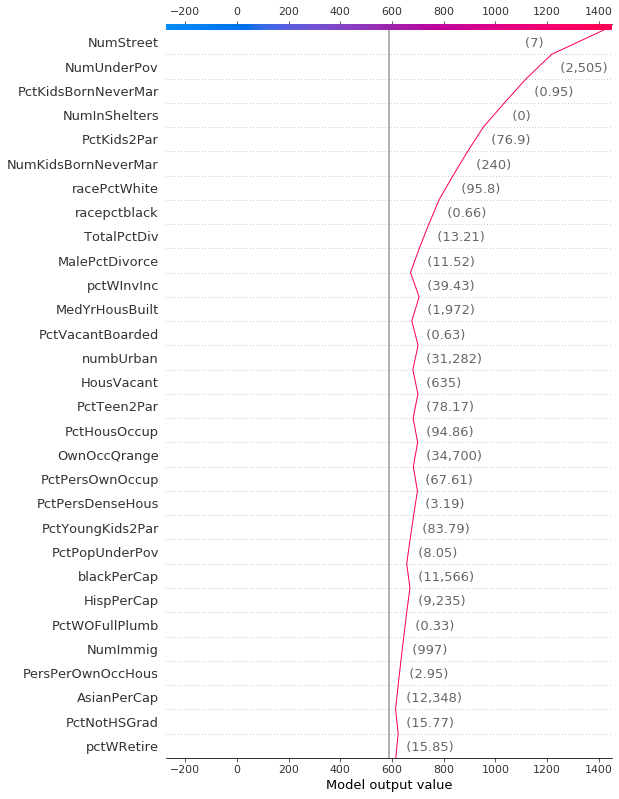## Visualize multioutput predictions¶ Decision plots can show how multioutput models arrive at predictions. In this example, we use SHAP values from a Catboost model trained on the UCI Heart Disease data set. There are five classes that indicate the extent of the disease: Class 1 indicates no disease; Class 5 indicates advanced disease. To keep the example short, the SHAP values are loaded from disk. The variable heart_base_values is a list of the SHAP expected values for each class. Likewise, the variable heart_shap_values is a list of SHAP matrices; one matrix per class. This is the multioutput format returned by shap.TreeExplainer. In : # Load all from disk to keep the example short. with open('./data/heart.pickle', 'rb') as fl: heart_feature_names, heart_base_values, heart_shap_values, heart_predictions = pickle.load(fl) class_count = len(heart_base_values)  Create a function that generates labels for the plot legend. Tip: Include the predicted values in the legend labels to help distinguish the classes. In : def class_labels(row_index): return [f'Class {i + 1} ({heart_predictions[row_index, i].round(2):.2f})' for i in range(class_count)]  Plot SHAP values for observation #2 using shap.multioutput_decision_plot. The plot's default base value is the average of the multioutput base values. The SHAP values are adjusted accordingly to produce accurate predictions. The dashed (highlighted) line indicates the model's predicted class. For this observation, the model is confident that disease is present, but it cannot easily distinguish between classes 3, 4, and 5. In : row_index = 2 shap.multioutput_decision_plot(heart_base_values, heart_shap_values, row_index=row_index, feature_names=heart_feature_names, highlight=[np.argmax(heart_predictions[row_index])], legend_labels=class_labels(row_index), legend_location='lower right')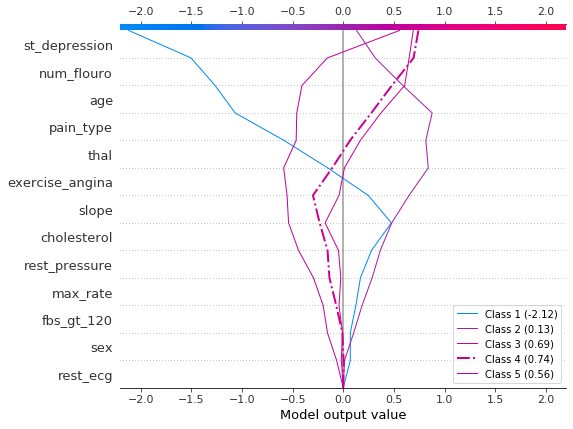For observation #3, the model confidently predicts that disease is not present. In : row_index = 3 shap.multioutput_decision_plot(heart_base_values, heart_shap_values, row_index=row_index, feature_names=heart_feature_names, highlight=[np.argmax(heart_predictions[row_index])], legend_labels=class_labels(row_index), legend_location='lower right')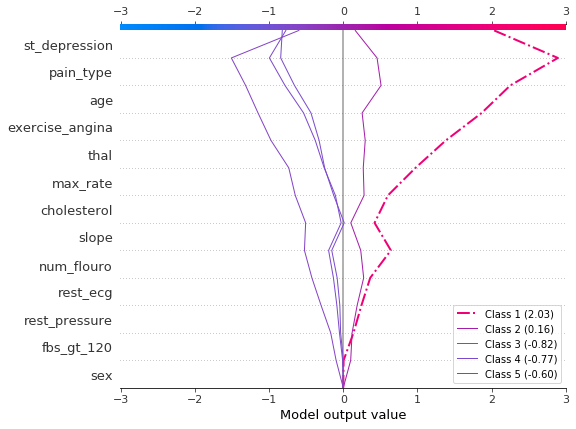## Display the cumulative effect of interactions¶ Decision plots support SHAP interaction values: the first-order interactions estimated from tree-based models. While SHAP dependence plots are the best way to visualize individual interactions, a decision plot can display the cumulative effect of main effects and interactions for one or more observations. The decision plot here explains a single prediction from the UCI Adult Income data set using both main effects and interactions. The 20 most important features are displayed. For more details relating to support for interactions, see the section "SHAP interaction values." In : shap.decision_plot(expected_value, shap_interaction_values[misclassified], features_display[misclassified], link='logit')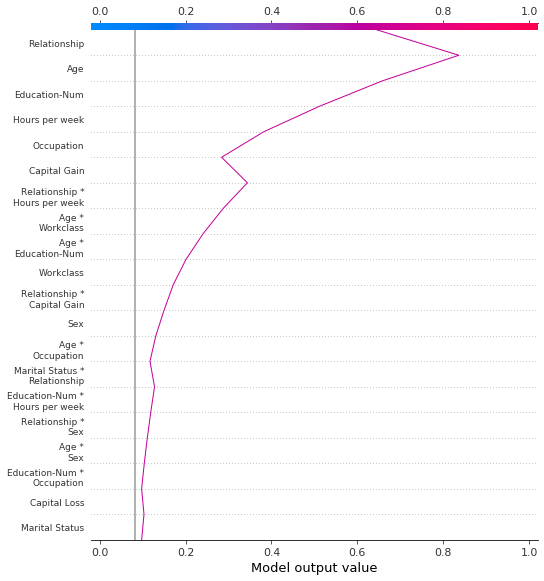## Explore feature effects for a range of feature values¶ A decision plot can reveal how predictions change across a set of feature values. This method is useful for presenting hypothetical scenarios and exposing model behaviors. In this example, we create hypothetical observations that differ only by capital gain. Start with the following reference observation from the UCI Adult Income data set. In : idx = 25 X_display.loc[idx]  Out: Age 56 Workclass Local-gov Education-Num 13 Marital Status Married-civ-spouse Occupation Tech-support Relationship Husband Race White Sex Male Capital Gain 0 Capital Loss 0 Hours per week 40 Country United-States Name: 25, dtype: object Create a synthetic data set using several copies of the reference observation. Vary the value of 'Capital Gain' from \$0-\\$10,000 by \$100. Retrieve the corresponding SHAP values. This approach allows us to evaluate and debug the model. Analysts may also find this method useful for presenting hypothetical scenarios. Keep in mind that the effects for capital gains shown in this example are specific to the reference record, and therefore cannot be generalized.

In :
rg = range(0, 10100, 100)
R = X.iloc[np.repeat(idx, len(rg))].reset_index(drop=True)
R['Capital Gain'] = rg

with warnings.catch_warnings():
warnings.simplefilter("ignore")
hypothetical_shap_values = explainer.shap_values(R)

hypothetical_predictions = expected_value + hypothetical_shap_values.sum(axis=1)
hypothetical_predictions = 1 / (1 + np.exp(-hypothetical_predictions))


This dependence plot shows the change in SHAP values across a feature's value range. The SHAP values for this model represent a change in log odds. This plot shows that there is a significant change in SHAP values around \$5,000. It also shows some significant outliers at \\$0 and approximately \\$3,000.

In :
shap.dependence_plot('Capital Gain', hypothetical_shap_values, R, interaction_index=None)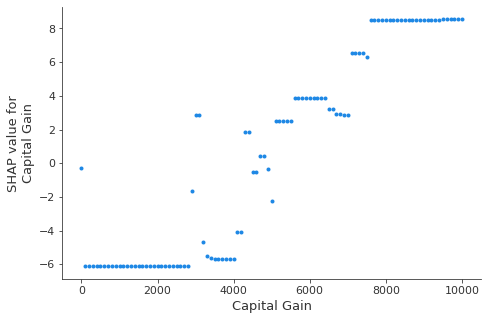Though the dependence plot is helpful, it is difficult to discern the practical effects of the SHAP values in context. For that purpose, we can plot the synthetic data set with a decision plot on the probability scale. First, we plot the reference observation to establish context. The prediction is probability 0.76. Capital gain is zero, for which the model assigns a small negative effect. The features have been ordered manually to match the next two plots.

In :
# The feature ordering was determined via 'hclust' on the synthetic data set. We specify the order here manually so
# the following two plots match up.
feature_idx = [8, 5, 0, 2, 4, 3, 7, 10, 6, 11, 9, 1]
shap.decision_plot(expected_value, hypothetical_shap_values, X_display.iloc[idx], feature_order=feature_idx,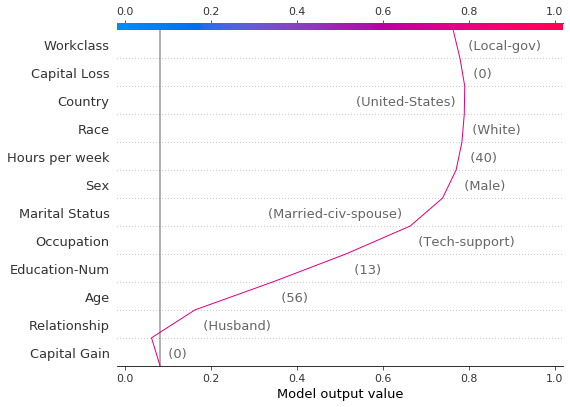shap.decision_plot(expected_value, hypothetical_shap_values, R, link='logit', feature_order='hclust', highlight=0)